top of page# Derivation of the Frequency Characteristics

The Natural Frequency

## Natural Frequency of a Spring-Mass Oscillator

### Spring-Mass Oscillator without Damping

The base of the spring-mass oscillator model is considered fixed in space. In the equilibrium position let x = 0. The mass is m [kg] and the spring constant is k [N/m].

To obtain an equation of motion one identifies all static and dynamic forces that occur. The weight force g   is invariant along x and in time and can therefore be left out.

The force acting on the mass due to the acceleration is .

The second force is caused by the spring and is k·x.

So for the equilibrium of the forces we writeorThis is a homogeneous differential equation.

For the solution one makes the ansatzThe characteristic equation iswith the solutionsUndamped spring-mass oscillatorWith                                         we find for the solution of the differential equationA and B are given by the initial conditions. If for instance the deflection or displacement x at time 0 is equal to X 0, then followsThis is a continuous, harmonic oscillation with the frequency ω₀.is called the natural frequency of the system.

### Spring-Mass Oscillator with Damping

We add a damping "dashpot" with the damping coefficient c.

The damping force is assumed to be proportional to the velocity and thus becomes c · .

The dimension of [c ] is N/m/s or kg/s.

The summation of all forces leads toHere it is useful to introduce the relative damping ζ which is defined asSpring-mass oscillator with damping

I.e. the damping is set in relation to m and ω0 . The "2" is an arbitrary factor.

With                        , the differential equation can be written in the formThe differential equation is homogenous again and we make the same ansatzThe characteristic equation is thenwith the solutionsFor ζ = 0, the result for the undamped oscillator is obtained again.
We are more interested here in the case of a weakly damped oscillator, i.e. ζ < 1. The root expression then becomes negative and the solutions of the characteristic equation will be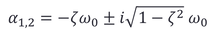They are conjugate complex
The total solution is equal to the sum of the particular solutions and becomesIf we set the imaginary partso we get

A and B are again determined by the initial conditions. With x(t=0)=0 for example, the solution is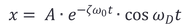This is a sinusoidal oscillation with the frequency ωD and the initial amplitude A.

The oscillation frequency ωis the damped natural frequency. Note that it is slightly different from ω0 of the undamped oscillation, depending on ζ .

## Frequency Characteristics of the Seismic Transducer

### Derivation of the Transfer Function

In contrast to the above we apply now a movement u=g(t) to the base. Thus, we force the oscillator to vibrate. The position of the mass m is given by u+x.

The acceleration force therefore becomesFor the equilibrium of the forces (we can leave out the static weight force again) we find the equationordividing by m , and introducing

and

we obtain the same differential equation as for the free oscillation, but now it is inhomogeneous due to the term on the rightSpring-mass oscillator under forced vibrationThe solution consists of the superposition of the solution of the homogeneous differential equation and a particular solution of the inhomogeneous differential equation.

Hence, we obtain a decaying first part, as in the case of free oscillation, as well as a second part, which follows the excitation u=g(t).

As excitation function we choose a harmonic oscillation (with constant displacement amplitude)with the derivations                                    and

The differential equation therefore becomesFor the solution we take the ansatzBy deriving and substituting one obtains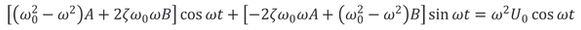A comparison of coefficients leads to the following system of equations for A and B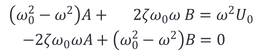The determinant of the coefficients is calculated as folows:The two solutions of the system of equations becomeand therforeWe transform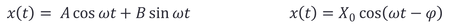into

For the amplitude and phase angle we getand thereby the final result of the

general transfer function:### Frequency Response of Displacement, Velocity and Acceleration

To make it easier and for transferable results it is better to use the frequency response representation. For the moment we only consider the amplitude response i.e. the ratio of the output amplitude to the input amplitude as a function of the frequency.

#### Displacement Frequency Response

Still with the output amplitude X0 and the input amplitude U0 we get from the general transfer function the amplitude responseWe introduce also the dimensionless relative frequency ωR  i.e. the excitation frequency in relation to the natural frequencyFor this we expand the expression of the frequency response with 1/ω0² to obtain the (dimensionless) displacement responseBoth the input U and output in our model above are displacements and the frequency response is therefore denoted by displacement amplitude response Φd.

The double logarithmic diagram shows the function for different values of the relative damping ζ .

We can see that for frequencies well above the natural frequency the transfer ratio becomes 1. This means in this region we have a 1 to 1Displacement amplitude response

displacement behaviour. Near to the natural frequency we find a more ore less pronounced amplification depending on ζ with a maximum atthe resonance frequency. Below the resonance the curve leads into slope of 40dB / decade.

#### Acceleration Frequency Response

For the frequency response with an acceleration signal as input we have to derive the input function twice.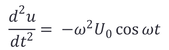The negative sign actually concerns the phase angle and we could also writeBecause we are not interested in the phase we can set it to zero and obtain for the

acceleration amplitude response Φ :The dimension of the function is [s²] because it is [displacement X/ acceleration Ü0 ].Acceleration amplitude response

Due to the double derivative the function now tilts to the other side. I.e. in acceleration terms we find a direct transfer in the frequency domain below the natural frequency, while we find a roll-off rate of -40dB per decade for high frequencies. The resonance region looks similar to the displacement response.

#### Velocity Frequency Response

Finally we can calculate and plot the amplification function for an input in velocity terms and we get the

velocity amplitude response Φv .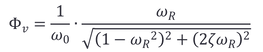The function is now symmetric around the natural frequency (logarthmic frequency axis).

The dimension of the function is

[displacement / velocity] = [s]Velocity amplitude response

#### Phase Response

The phase response is the same in all three cases, displacement velocity and acceleration.The change in phase through the resonance must not be confused with the fact that displacement, velocity and acceleration are each offset by π/2.Phase angle frequency response

### Resonance Frequency

In sensor technology, the resonance is defined as the maximum of the output amplitude for a constant imput amplitude.

Therefore, in view of the three different amplitude response functions, we also obtain different resonant frequencies for displacement, velocity and acceleration. We can obtain the values by deriving the function Φ  and setting it to 0.

For the acceleration resonance as an example we obtainand we find the zero atThus one obtains for the different resonance frequencies in an analogous way:

Acceleration resonance frequency:Velocity resonance frequency:Displacement resonance frequency:#### How can it be that you get three different solutions for the same physical phenomenon?

At the resonance frequency, the oscillator absorbs the maximum power. Since the power is equal to force times velocity, this maximum is found when the phase angle of the output signal x is equal to π/2. In this situation, the amplitude of the system increases continuously until the energy absorption is in equilibrium with the energy loss due to damping.
This means that the physical phenomenon of the resonance is always at a phase angle of π/2 and this is always the case at ω= ω0 .
So in order to see the "correct" (physical) resonance we need to keep the velocity amplitude of the input signal constant. We note that then the resonance is at ω0.
With constant acceleration, the velocity amplitude decreases with increasing frequency with the factor 10 per decade. This means that the energy applied becomes greater at frequencies below ω0  and therefore the maximum-amplitude resonance shifts downwards.

The same, but in opposite, is true for constant displacement. Here, the maximum-amplitude resonance is shifted upwards.

Frequency Characteristics
Resonance Frequency
bottom of page